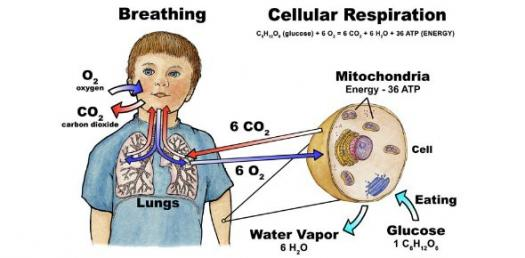Introductions Of Cellular Respiration

5 QuestionsSettingsCan you correctly answer all these questions on cellular respiration? Let's find out now by taking this quiz!

• 1.
Cellular respiration takes place in...
• A.

Animals

• B.

Plants

• C.

Fungi

• D.

All of the above

• 2.
Is  C6H12O6 + 6 O2 --> 6 CO2 + 6 H2O the equation for cellular respiration?
• A.

True

• B.

False

• 3.
What breaks down during cellular respiration?
• A.

Glucose

• B.

Carbon dioxide

• C.

Glucose and oxygen

• D.

Oxygen and carbon dixide

• 4.
The cell does not use...
• A.

Carbon dioxide

• B.

Energy

• C.

Oxygen

• D.

Glucose

• 5.
The cell keeps...
• A.

Sugar

• B.

Energy

• C.

Glucose

• D.

Oxygen

Related Topics